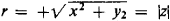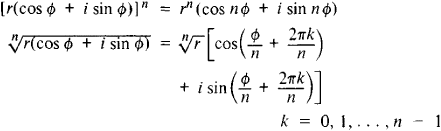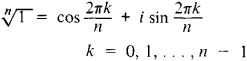# Complex Numbers

Also found in: Dictionary, Thesaurus.
Related to Complex Numbers: Imaginary numbers
The following article is from The Great Soviet Encyclopedia (1979). It might be outdated or ideologically biased.

## Complex Numbers

numbers of the form x + iy, where x and y are real numbers and i is the so-called imaginary unit (a number whose square equals — 1); x is called the real part and y the imaginary part of the complex number z = x + iy (x = Re z, y = Im z). Real numbers are a particular case of complex numbers (y = 0). Complex numbers that are not real (y ≠ 0) are called imaginary numbers. A complex number with x = 0 is called pure imaginary. The complex numbers z = x + iy and z = x — iy are called complex conjugates. Arithmetic operations on complex numbers are carried out according to the usual rules of operation on polynomials with the added condition that i2 = — 1. Every complex number x + iy can be geometrically depicted as a point on a plane with rectangular coordinates x and y (see Figure 1). If the polar coordinates of this point are denoted by r and Φ, then the corresponding complex number may be represented in the form

r(cos ø + i sin ø)

(the trigonometric, or polar, form of a complex number);is called the modulus of the complex number x + iy, and Φ = arg z is called its argument. The trigonometric form of complex numbers is especially convenient for the operations of involution and evolution:In particular,In its algebraic properties the set of complex numbers forms a field. The field is algebraically closed, that is, any equation xn + a1xn-1 + . . . + an = 0, where a1 . . . , an are complex numbers, has exactly n roots (not necessarily distinct) among the complex numbers.Figure 1

Ancient mathematicians had encountered the extraction of square roots of negative numbers in the process of solving certain problems; in these cases the problems were considered unsolv-able. But when formulas for solving cubic equations were found in the first half of the 16th century, it turned out that in the irreducible case real roots of equations with real coefficients are obtained as a result of operations on complex numbers. This contributed to the acceptance of complex numbers. The first demonstration of the simplest operations with complex numbers is found in a work by R. Bombelli (1572). However, for a long time complex numbers were considered as something supernatural. Thus, G. von Leibniz wrote in 1702: “The divine spirit found a sublime outlet in that wonder of analysis, that portent of the ideal world, that amphibian between being and not-being, which we call the imaginary root of negative unity.” In 1784, L. Euler discovered the wonderful formula e = cos Φ + i sin Φ, which was the first important result of the theory of functions of a complex variable; however, the true nature of complex numbers was revealed only at the end of the 18th century when their geometric interpretation was discovered (see above).

The term “complex number” was proposed by K. Gauss in 1831. The introduction of complex numbers—an important stage in the development of the concept of number—has made many mathematical investigations clearer and more uniform. Complex numbers are now used in mathematical descriptions of many problems in physics and technology (hydrodynamics, aeromechanics, electrical engineering, atomic physics). The principal branches of classical mathematical analysis acquire total clarity and completeness only with the use of complex numbers, which explains the central place occupied by the theory of functions of a complex variable. (SeeANALYTIC FUNCTIONS.)

### REFERENCES

Markushevich, A. I. Kompleksnye chisla i konformnye otobrazheniia, 2nd ed. Moscow, 1960.
Kurosh, A. G. Kurs vysshei algebry, 9th ed. Moscow, 1968.
References in periodicals archive ?
As well as the electricity that flows along solid metal conductors, systems that use invisible electromagnetic waves that travel through the air and the vacuum of space and designed with the help of complex numbers. These systems include radios, televisions, microwave ovens, infrared remote controls, lasers, UV lighting, X-rays and gamma waves to help kill cancers in people.
where a, [b.sub.1], [b.sub.2], ..., [b.sub.r] are real numbers, and in this case [N.sub.r] is called a refined neutrosophic real number; and if at least one of a, [b.sub.1], [b.sub.2], ..., [b.sub.r] is a complex number (i.e.
By the definition of generalized m-power matrix in , we know that a generalized mpower matrix A is a one which satisfies that [[product].sup.m.sub.i = 1](A + [[lambda].sub.i]I) = 0, where [[lambda].sub.1], [[lambda].sub.2], ..., [[lambda].sub.m] are the pairwise different complex numbers. Note that I is invertible and commutative with A.
We add complex numbers by adding the real and imaginary parts separately.
Theorem 2 Let x, y be complex numbers such that [[absolute value of x].sup.2]+ [|y|.sup.4] < 1.
The solutions of complex equations (1 and 2) are complex numbers, i.e.
Rather than demonstrating flexible thinking and responding to the new mathematical context of complex numbers, this student is attached to the 'common denominator' form to 'simplify' fractions.
Let a be a complex number and let q be a univalent function such that zq'(z)/[(q(z)).sup.[alpha]] is starlike in E.
Show that the product of the complex numbers a + bi, a - bi (where a, b are real), is a real number.
Certainly amplitudes for the particle traveling along both path 1 and 5, say, exist, but amplitudes are just complex numbers. They are not states.
Though the book advises readers to have a background in physics and calculus and a working knowledge of complex numbers and vectors, physicist McMahon clearly defines the notions of blackbody radiation, the photoelectric effect, the Schrodinger equation, and vector space.
It supports 1999 ISO C Standard complex numbers, variable-length arrays, and the Institute of Electrical and Electronic Engineers' 754 standard for floating-point arithmetic and generic mathematical functions.

Site: Follow: Share:
Open / Close